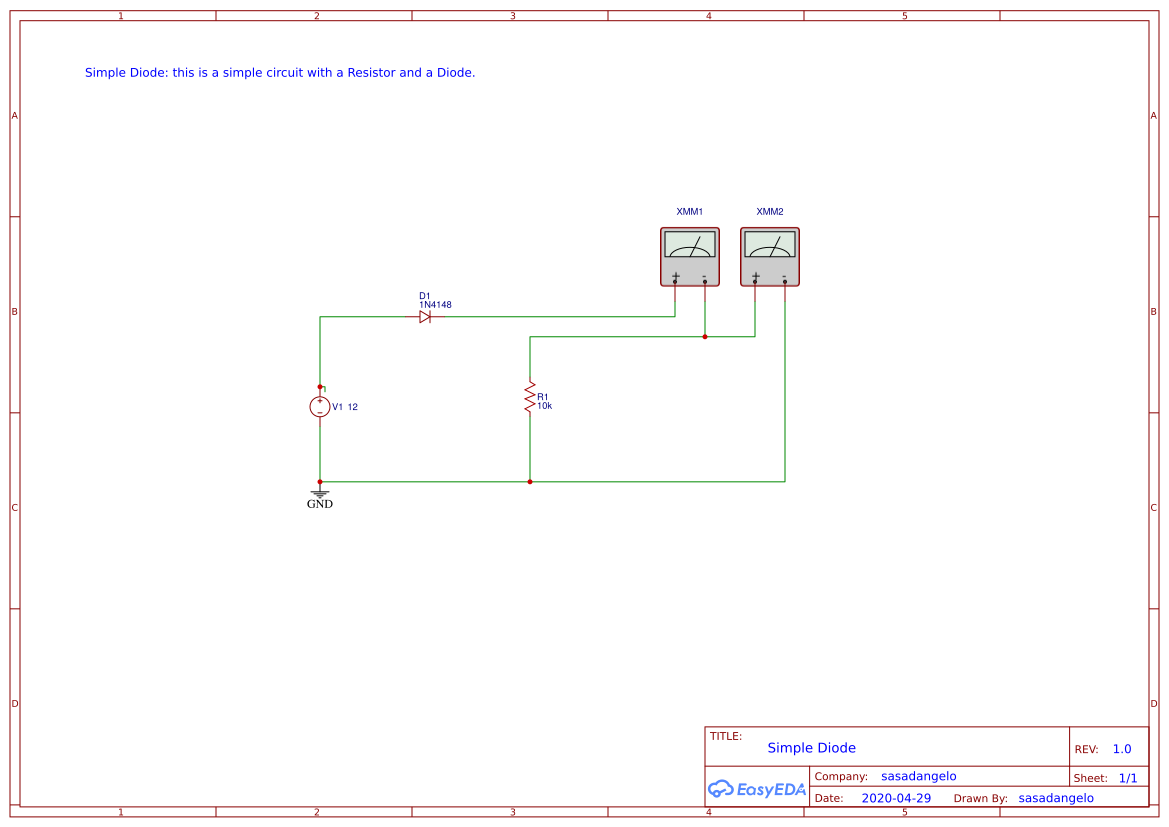Public Domain

##### Tags
Recommend Projects

# Simple Diode

2 months ago 40
• Description
• Documents
• BOM
• Attachments
• Members

## Description

This is a simple project with a Power Supply, a Diode, and a Resistor. The goal of this project is simply to test my old electronic knowledge. Around the web, there are a lot of resources that explain how a diode works, for example: https://learn.sparkfun.com/tutorials/diodes/all

I connected a 12V DC battery to a diode connected in series to a 10k Resistor. There are some multimeters just to check the current that passes and the voltage on the resistor (Vd=12V-Vr).

I used a 1N4148 diode, datasheet here: https://www.diodes.com/assets/Datasheets/ds12019.pdf

Looking at the datasheet we see that this diode requests at least a 0.62 V to start flows and a max of 1V for 300mA of current. I tested the circuit with different resistors and Vd changed in a range of 0.6-0.9V, so in general, it is convenient to consider Vd=0.7V on average.

With a good approximation: Vr=V-Vd Ir=Vr/R

To make calculus more precise, when the resistor is:

• < 1k we can consider Vd=0.8
• 1k < R < 10k we can consider Vd=0.7
• >10k we can consider Vd=0.6

## Tests with different Resistors

R=10k Vd=0.6 Ir=1.14 mA Vr=11.51V

R=1k Vd=0.7 Ir=11 mA Vr=11.3V

R=0.5k Vd=0.77 Ir=23 mA Vr=11.26V

R=100 Vd=0.86 Ir=111 mA Vr=11.13V

## Simple Diode## BOM

ID Name Designator Quantity
1 12 V1 1
2 Multimeter XMM1,XMM2 2
3 10k R1 1
4 1N4148 D1 1

## Attachments

None
Success
The owner does not allow comments in this project now